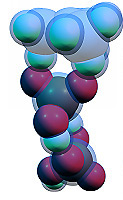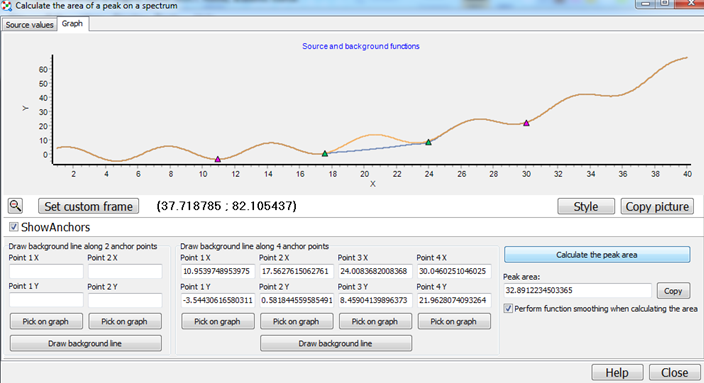## Peak area calculator

This window provides a tool for quick calculation of areas of peaks on custom graphs:For using this utility, you should copy your function in text format, then switch to the Graph tab and choose the anchor points which define your peak. You can choose either 2 or 4 anchor points and then press the button "Draw background line" to build the background line. After pressing one of the "Pick on graph" buttons, you should click on the graph to place the anchor point. For 2 points, the background line will be drawn as a simple line between these two points; for 4 points, Chemcraft will build a 3-degree polynom around these 4 points.

After pressing the "Calculate the peak area" button, Chemcraft will compute the area of the peak as the difference between the integral of you curve and the integral of the background line.

Algorithm

If the checkbox "Perform function smoothing when calculating the area" is checked, Chemcraft divides each interval between two close points of your curve into 20 smaller intervals, and draws a spline between these points. When the functions are integrated, each point is simply considered as a rectangle with the height of the Y value and the width of the x step; this is drawn on the graph so the area what is calculated is equal to the area which you sea on the graph.

BACK